Ex 15.1

Chapter 15 Class 10 Probability (Term 1)
Serial order wise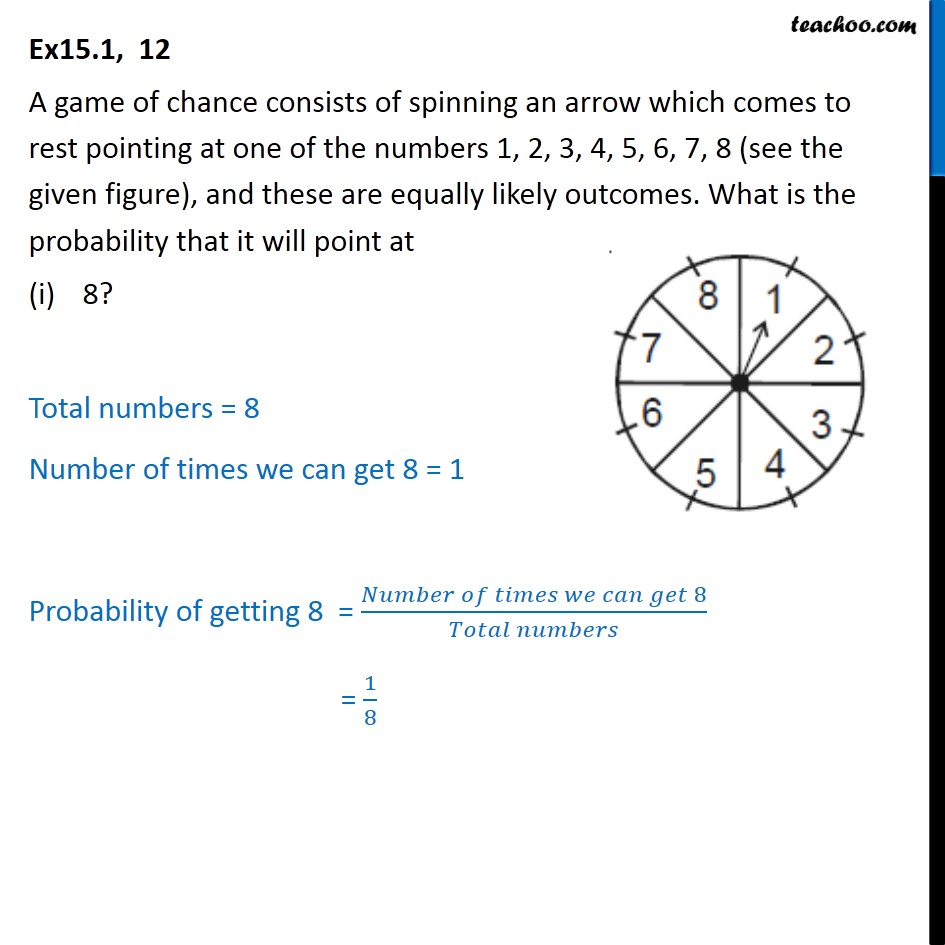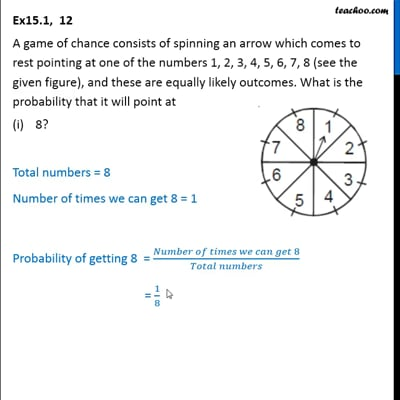This video is only available for Teachoo black users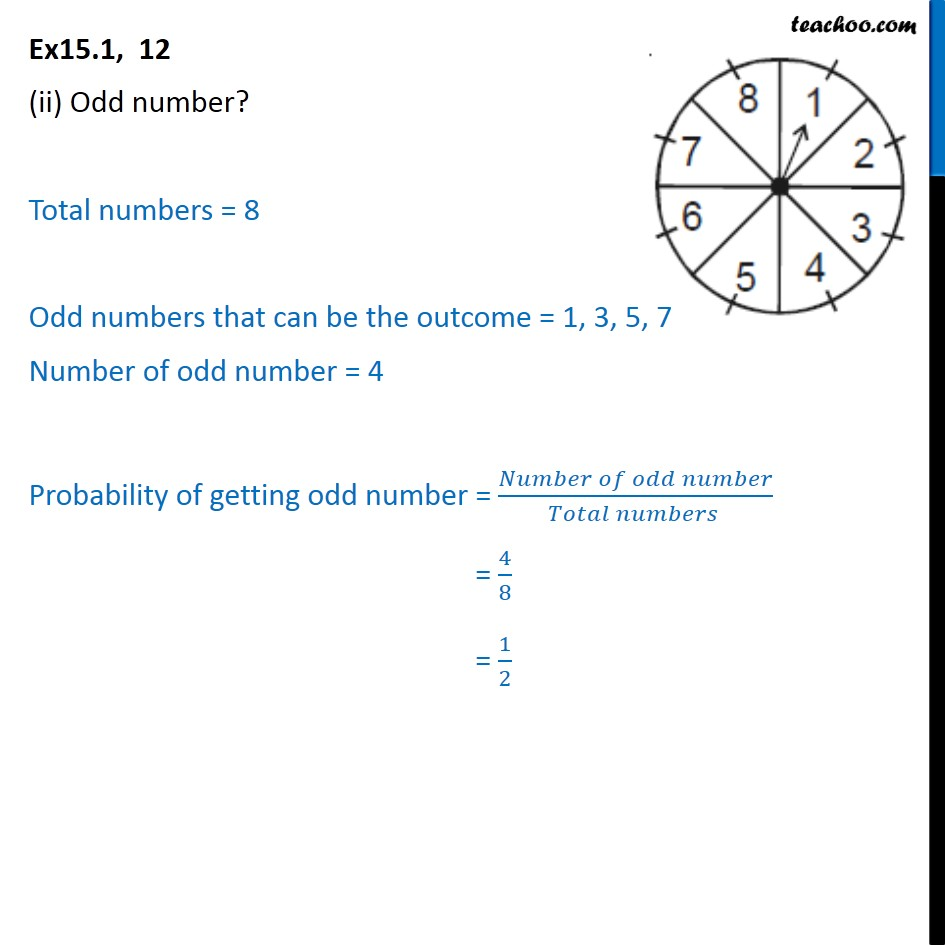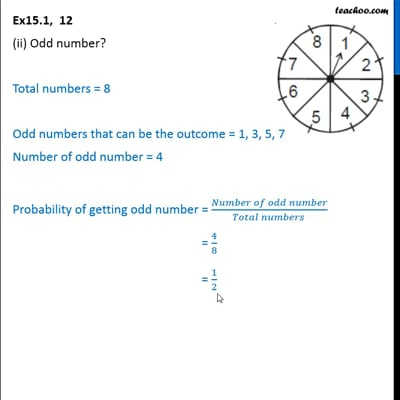This video is only available for Teachoo black users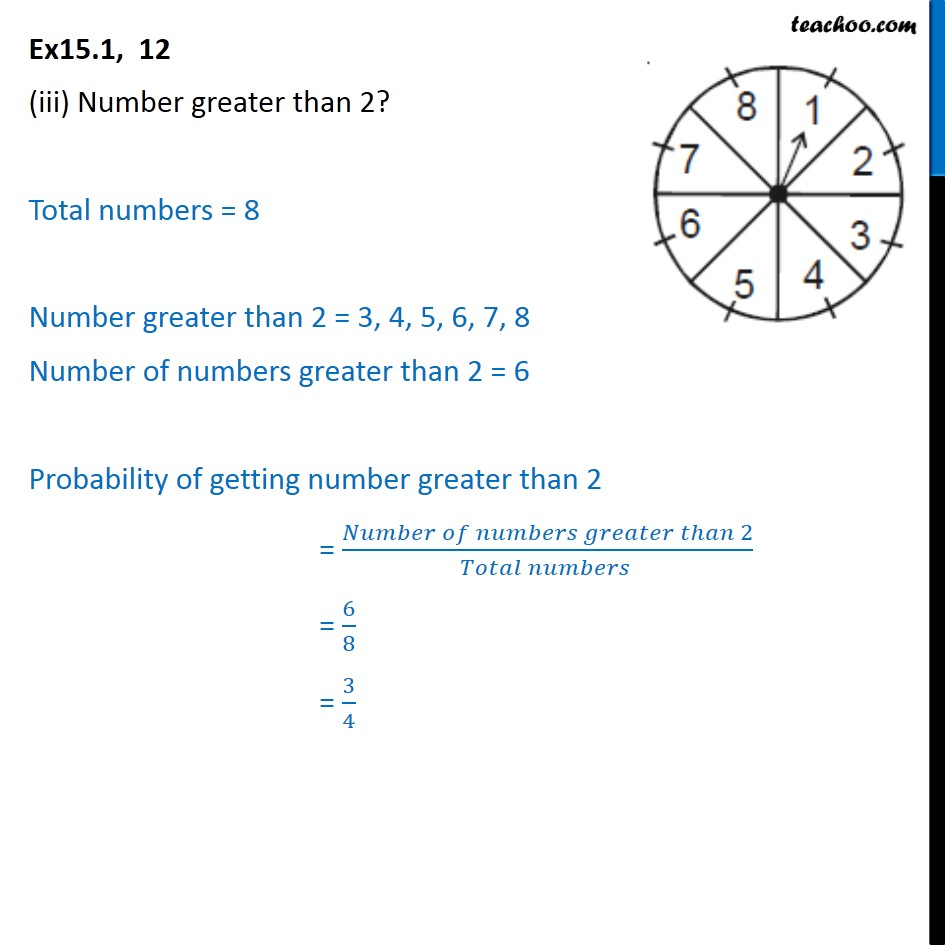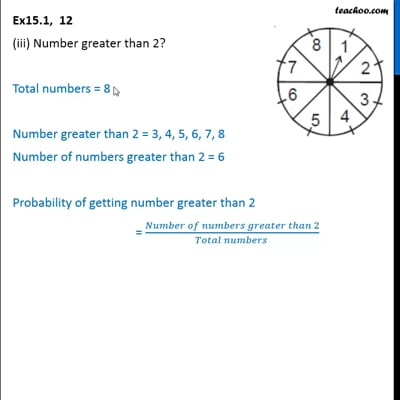This video is only available for Teachoo black users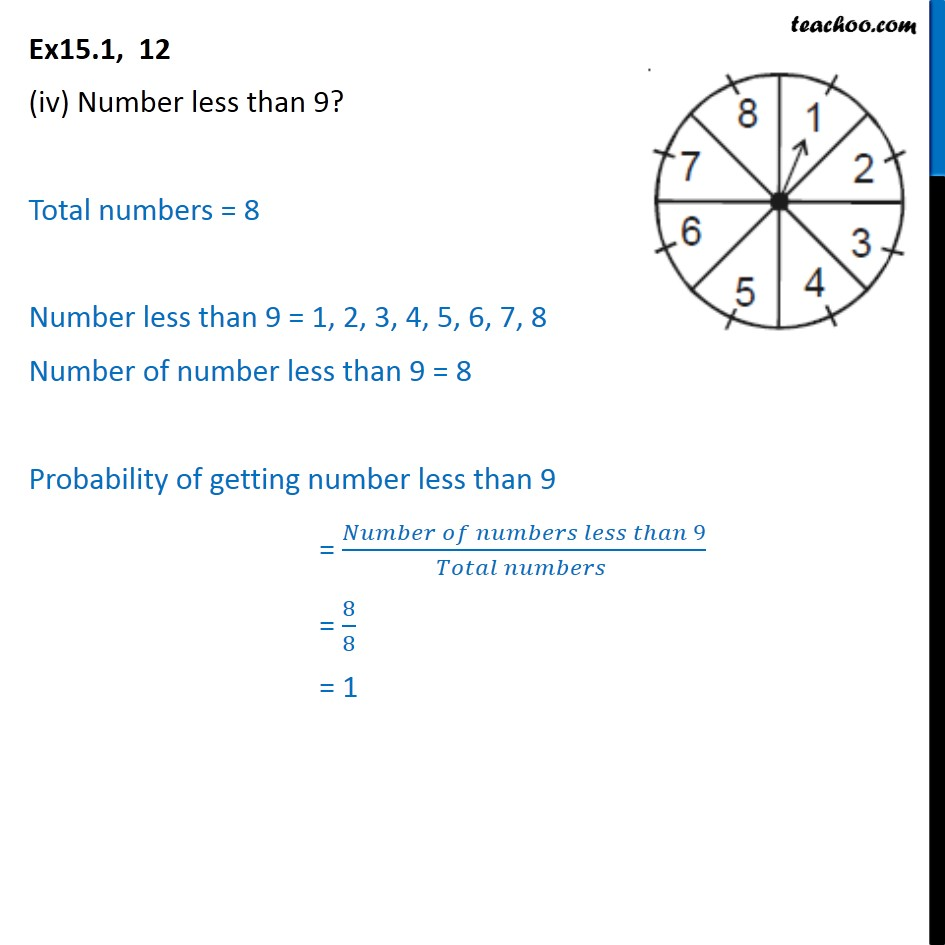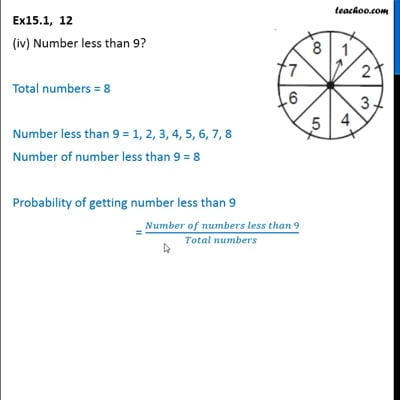This video is only available for Teachoo black users

### Transcript

Ex15.1, 12 A game of chance consists of spinning an arrow which comes to rest pointing at one of the numbers 1, 2, 3, 4, 5, 6, 7, 8 (see the given figure), and these are equally likely outcomes. What is the probability that it will point at 8? Total numbers = 8 Number of times we can get 8 = 1 Probability of getting 8 = ( 8)/( ) = 1/8 Ex15.1, 12 (ii) Odd number? Total numbers = 8 Odd numbers that can be the outcome = 1, 3, 5, 7 Number of odd number = 4 Probability of getting odd number = ( )/( ) = 4/8 = 1/2 Ex15.1, 12 (iii) Number greater than 2? Total numbers = 8 Number greater than 2 = 3, 4, 5, 6, 7, 8 Number of numbers greater than 2 = 6 Probability of getting number greater than 2 = ( 2)/( ) = 6/8 = 3/4 Ex15.1, 12 (iv) Number less than 9? Total numbers = 8 Number less than 9 = 1, 2, 3, 4, 5, 6, 7, 8 Number of number less than 9 = 8 Probability of getting number less than 9 = ( 9)/( ) = 8/8 = 1